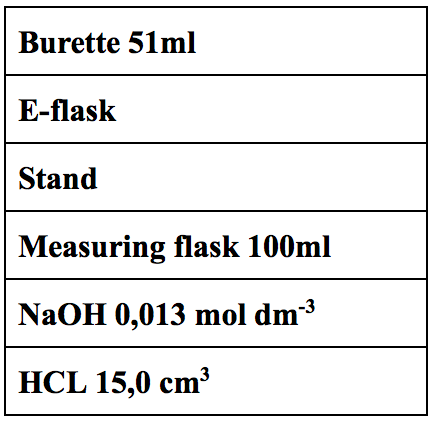Search

# Titration of HCL with NaOHPractical report - Titration of hydrochloric acid with Sodium Hydroxide

Caution: Hydrochloric acid, as well as Sodium Hydroxide, are both very strong acid/base and harmful to skin and eyes. If any contact to the human body would occur, that section of the body needs to be washed thoroughly with a good amount of water and taken to the emergency room if necessary.

The purpose : The purpose of this practical experiment is to go through the process of neutralization reaction with the acid HCl and Base NaOH.

Background information

The practical was an acid-base neutralization titration in which HCL (acid) and NaOH (base) were used in the experiment. (1, 2) A titration is a chemical technique in which a reagent called a “Titrant” of known concentration also called a standardized solution is used to determine the concentration of an analyte or unknown concentration of a known concentration. Considering the fact that we know what the chemical is, we will know how it will react and thus we can use the reaction to determine the concentration of the solution. (2) In this case, we have an unknown concentration of acid, we can use a known concentration of hydroxide base and this type of action is called a neutralization reaction, where salt and water are products of the reaction.

Acid + Base ⇒ Salt + Water

HCL + NaOH ⇒ NaCL + H2O

We will use BTB, which is a chemical pH indicator that will changes color depending on pH changes to show us when the solution has been fully neutralized. It is good to consider that BTB on its own is a bit acidic which is why when it is added to HCL, the solution turns yellow. (3) The point in which all the acid will be absorbed and no excess base will remain in the solution is called the equivalence point. When the equivalence point will be reached, we will be able to use that state of the solution to determine the initial concentration of acid using a series of calculations. The goal of the titration is to reach as close as possible to the equivalence point by carefully adding the base, which will ensure that the calculated acid concentration is as close to the true value of neutralization reaction as possible. In this case, three titration tries were made until we reached the point of neutralization reaction, where the solution turned green.

MaterialsMethod

Three titration processes were completed with the final one being a success. Thus meaning that even if the solution of the base is 1%greater than the acid, the experiment becomes a fail and another trial needs to be done. If too much base will be added to the acid, the solution will turn blue, but just the right amount will turn the solution green. The experiment was set up with a stand holding the Burette with its clip, whilst the E-flask was set directly under on a stable table  37 dm3 of NaOH was poured directly into the ±0.1cm3 Burette as well as 15,00 cm3 was measured with a 100 ml ±0.1cm3 measuring cylinder and then poured into the 200 ml ±0.5 cm3 E-flask  First trial began with rough titration, where fast drops of NaOH were dropped straight into HCL and we saw a failed trial around 25 ml of NaOH in HCL  The blue solution was poured into a separate E-flask and the Burette was filled up to 37 ml of NaOH again  Second trial began with a much slower titration with approximately 1 drop each second and the experiment failed again at 25 ml of NaOH again  The blue solution was poured into a separate E-flask and the Burette was filled up to 37 ml of NaOH again  The third trial was a success with approximately 1 drop each 2 seconds with a more patient titration where the equivalence point was reached when only 24.4 ml of NaOH was left in the Burette.

ResultCalculations :

24,4 ml - 12 ml = 12,4 ml ⇒ Neutral

49 - 12 cm3 = 37 cm3 of NaOH

or

We ended with 24,4 ml of NaOH

37 - 24,4 = 12,4 into the acid HCL

NaOH + HCL ⇒ NaCL + H2O

Mol ratio = 1:1:1:1

V(NaOH) = 0,012 dm3

C(NaOH) = 0,013 mol dm3

n(NaOH) = 0,013 x 0,012 = 1,56 x 10-4

C = n/V ⇒ 0,000156/0,015 = 0,0104 mol dm-3

Conclusion and evaluation

Reference :

1. Chemistry Libretexts. Titration. Available from: https://chem.libretexts.org/Core/Analytical_Chemistry/Lab_Techniques/Titration [Accessed 10th May 2017]

2. Spark-notes. Acid-Base Titration. Available from: http://www.sparknotes.com/chemistry/acidsbases/titrations/section1.rhtml [Accessed 11th May 2017]

3. Khan Academy. Acid-Base titration curves. Available from: https://www.khanacademy.org/test-prep/mcat/chemical-processes/titrations-and-solubility-equilibria/a/acid-base-titration-curves [Accessed 12th May 2017]

4. Titrations.info. Titration and titrimetric methods. Available from: http://www.titrations.info/titration-errors [Accessed 20th May 2017]